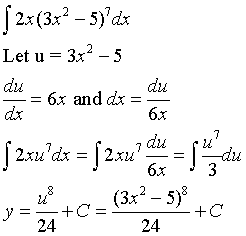# Integration by u-substitution

• b_roberts

## Homework Statement## The Attempt at a Solution

I just started integration. This is the first example from the lesson. I get to the part where they take the derivative of u with respect to x. But the next step, where it says dx=du/6x, I'm totally lost. I don't understand how they got that, or what it means, or even how it is read.

du/dx=6x

basic algebra rules ofmultiplying by dx and then dividing by 6x gives us dx.

Basic rules of algebra don't apply -- this is a change of variable in an integral substitution (or maybe algebra of differential forms, depending on your POV). Happily, the result is similar to basic rules of algebra, so it's easy to remember/manipulate.

How can you multiply and divide to get dx=du/6x if du/dx is a symbol of notation and has nothing to do with division? I just want to understand where the dx = du/6x comes from, and how it is supposed to be read. Is it read "with respect to x = the derivative of u divided by 6x?

In the integration when you do u sub, you still have a dx. How can you integrate variable u with a dx?

In order to do so, you need to know what dx is in u.

To find that, you take (du/dx)*dx=6x*dx which yields du=6x*dx so now we divide. du/6x=(6x*dx)/6x. Now we have dx=du/6x.

Lastly, subsitute back in so you have an integral that is in terms of u only.

I don't understand how you can multiply du/dx by dx and then cancel the dx from the numerator and denominator, though. I thought du/dx was a symbol of notation?

How is one suppose to integrate u with a differential of dx?

I don't understand how they got that, or what it means, or even how it is read.

dx and du are read how they look like, e.g. dx --> [Dee Ex]. And from du/dx=6x to dx=du/6x, think about the definition of a derivative. $$dy/dx=\underbrace{lim}_{\Delta x\rightarrow 0}\frac{\Delta y}{\Delta x}$$

Just like $$\Delta y$$ and $$\Delta x$$, dy and dx can be separated. Think of a curve, the slope of a tangent line at an arbitrary point can be represented by rise/run. Actually draw the rise and run, you will get dy as the rise and dx as the run.

This may help: when you do sub, you don't have to change the variables. Instead we can change the differential.

Your new dx would be (6xdx) as we see there is a x in the integral and an x in the differential which is what we needed. Since we now have a multiple of 6, we need to divide by 6 to make up for the difference.

The new integral is now 2/6 int (x(3x2-5)7)(6xdx). 2/6 since I brought the 2 out of the integral.

Now when you integrate just integrate this, (3x2-5)7), as one term.

I don't understand how you can multiply du/dx by dx and then cancel the dx from the numerator and denominator, though. I thought du/dx was a symbol of notation?
It is, and you aren't really canceling the dx's. But as Hurkyl said above, everything works as if you can. Think of it as a mnemonic device for changing variables in an integral.